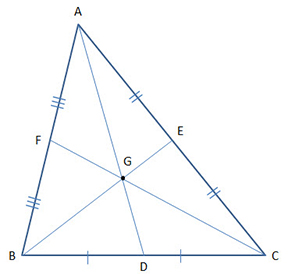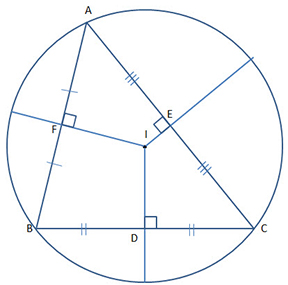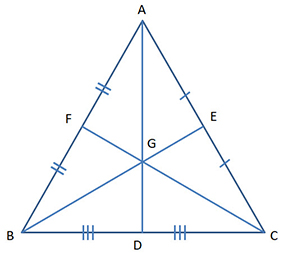Centroid

The point of intersection of the three medians is called the centroid of the triangle.

In the adjoining figure,$G$ is the centroid.$AP, BQ \ and \ CR$  are the medians which divide the corresponding sides$BC, AC \ and \ AB$  respectively in two equal halves.

Hence,$BP=PC, CQ=QA \ and \ AR=RB$

The centroid of the triangle always divide each of the medians in the ratio of$2:1$

Therefore,$AG:GP = 2:1,$$BG:GQ=2:1 \ and \ CG:GR=2:1$$\\$

Incentre

The point of intersection of the bisectors of the internal angles of a triangle is called the Incentre of the triangle.The Incentre of the triangle is equidistant from each of the sides of the triangle.

Hence$IF=IE=ID$

If you draw a circle, with$I$ as the center, then the radius of this Incircle would be$IF \ or \ IE \ or \ ID$

In the adjoining figure,$AI, BI \ and \ CI$  are bisectors of angles$A, B \ and \ C$  respectively.$\\$

CircumcenterThe point of intersection of the perpendicular bisectors of the three sides is the circumcenter of the triangle. In the adjoining diagram, you can see that$BD=CD, CE=AE \ and \ AF=CF$.

The distance from the center to the three vertices$A, B \ and \ C$  are equal. i.e.$AO = BO=CO$

If you draw a circle with$O$  as the center, and the radius$AO \ or \ BO \ or \ CO$, the circle will encircle the triangle and touch the three vertices.$\\$

OrthocenterThe point where the three perpendiculars drawn from the vertices of a triangle to the opposite side of the triangles meet is called the orthocenter of the triangle.

In the adjoining figure,$AD \perp BC, AD \perp BE \ and \ CF \perp AB$$\\$

Properties of Isosceles TriangleIf a triangle is an Isosceles triangle, then

Median$AD = bisector of \angle A$

= perpendicular bisector of opposite side$BC$

= Altitude of corresponding side$BC$$\\$

Properties of Equilateral Triangle

If the triangle is an equilateral triangle, thenMedian$AD =$  bisector of$\angle A$

= perpendicular bisector of opposite side$BC$

= Altitude of corresponding side$BC$

Median$BE =$  bisector of$\angle B$

= perpendicular bisector of opposite side$AC$

= Altitude of corresponding side$AC$

Also if$G$  is the centroid of the triangle, it is also the Incentre, it is also the circumcenter and also the orthocenter.